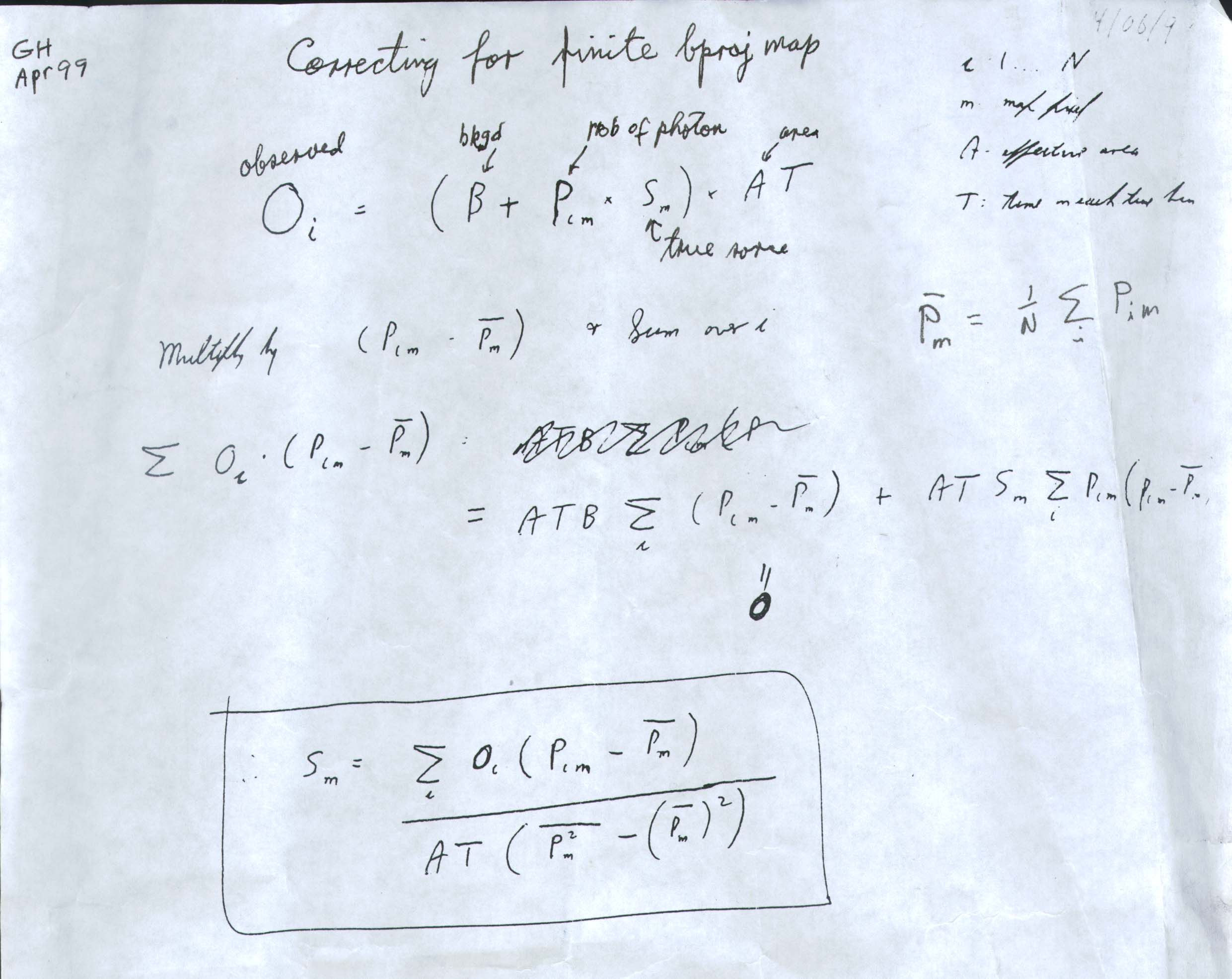# Back-Projection Corrections

Earlier in 1999, Gordon Hurford derived an equation for correcting the back-projection images. This is called "flat-fielding" in the HESSI software. The equations were taken from a derivation by Durouchoux et al (1983) quoted here:

# Gamma Ray Imaging with a Rotating Modulator

Astronomy And Astrophysics 120, 150-155 (1983)
• P. Durouchoux
• H. Hudson
• G. Hurford
• K. Hurley
• J. Matteson
• E. Orsal
[ Excerpt from Section 2]

Any modulator/detector configuration may be evaluated by considering the three following properties: sensitivity, angular resolution, and selectivity. Each of these may be defined in terms of its vector interpretation or its physical interpretation (See Table 1). That the sensitivity in a given pixel is proportional to the square root of the variance of the sky vector for that pixel may be shown as follows. Suppose that a single point is observed in pixel j which would cause each detector to have a constant counting rate s counts/s if no other modulator was present, while the background counting rate, assumed constant on the average from detector to detector, is b counts/s. If the observation lasts a time T seconds,

Oi = (b + s Si)T,

In practice, the source strength s may be extracted from the observation vector O by first multiplying through by a function whose average is zero, namely

Sji-<Sj>,

where <Sj> = Σi(Sji)/Nc

Then, summing over the i components of the vector, the background contribution drops out:

Σi[Oi(Sji-<Sj>)] = Σi[bT(Sji-<Sj>)] + Σi[sTSji(Sji-<Sj>)]

= sTNc(<Sj2> - <Sj>2)

The standard deviation associated with the number of counts in the ith component is. for Gaussian statistics,

(Oi)½ = ({b+sSji}T)½

Since the standard deviations add as the square root of the sum of the squares, the standard deviation associated with the above is

i[Oi(Sji-<Sj>)2] )½

= Nc½i[bT(Sji-<Sj>)2]+ Σi[sTSji(Sji-<Sj>)2])½

=(bTNc)½ ((<Sj2> - <Sj>2))½

for b >> s. Thus the statistical significance of the observation is

s(TNc/b)½ ((<Sj2> - <Sj>2))½

or just proportional to the square root of the variance of the sky vector.

Notes transcribed from GH.Notes by GH, April 1999

Oi =(B + Pim Sm) AT(1)

Oi=observed counts in time bin i
B=background (assumed constant)
Pim=probability of photon passage at pixel m, time i
Sm=true source strength at pixel m (presumed a point source?)
A=effective area of collimator
T=time in time bin i (assumed equal for all time bins)
i=1,...,N

from which GH derived:

Sm= Σi Oi ( Pim - <Pm >)/ {AT ( < Pm2 > - <Pm >2)}(2)

where < Pm 2 > is the mean square of Pim, averaged over time, and <Pm >2 is the squared mean, averaged over time.

It appears from equation (1) that the point source Sm is multiply determinate. That is, for each i and fixed m, there are N equations which determine Sm.

The expressions for the point source strength in back projection and clean.pro (also MEM?) must be replaced with the time-averaged values of equation (2) for Sm.

Edward J. Schmahl